# NCERT Exemplar Class 12 Chemistry Solutions for Chapter 9 - Coordination Compounds

NCERT Class 12 Chemistry Exemplar Solutions for Chapter 9 Coordination Compounds comprise questions taken from the NCERT Exemplar Class 12 Chemistry book, along with a few questions framed by the subject experts of BYJU’S, questions from previous years’ question papers and sample papers, numerical problems and worksheets. Getting well-versed with the exemplar questions will equip students to face the Class 12 Chemistry examination and help them score good marks in graduate entrance examinations, like JEE and NEET. The NCERT Class 12 Chemistry Exemplar for Chapter 9 PDF provides you with various questions, such as MCQs, short answer questions, numerical problems, match the following and fill in the blanks.

## Download the PDF of NCERT Exemplar Solutions for Class 12 Chemistry Chapter 9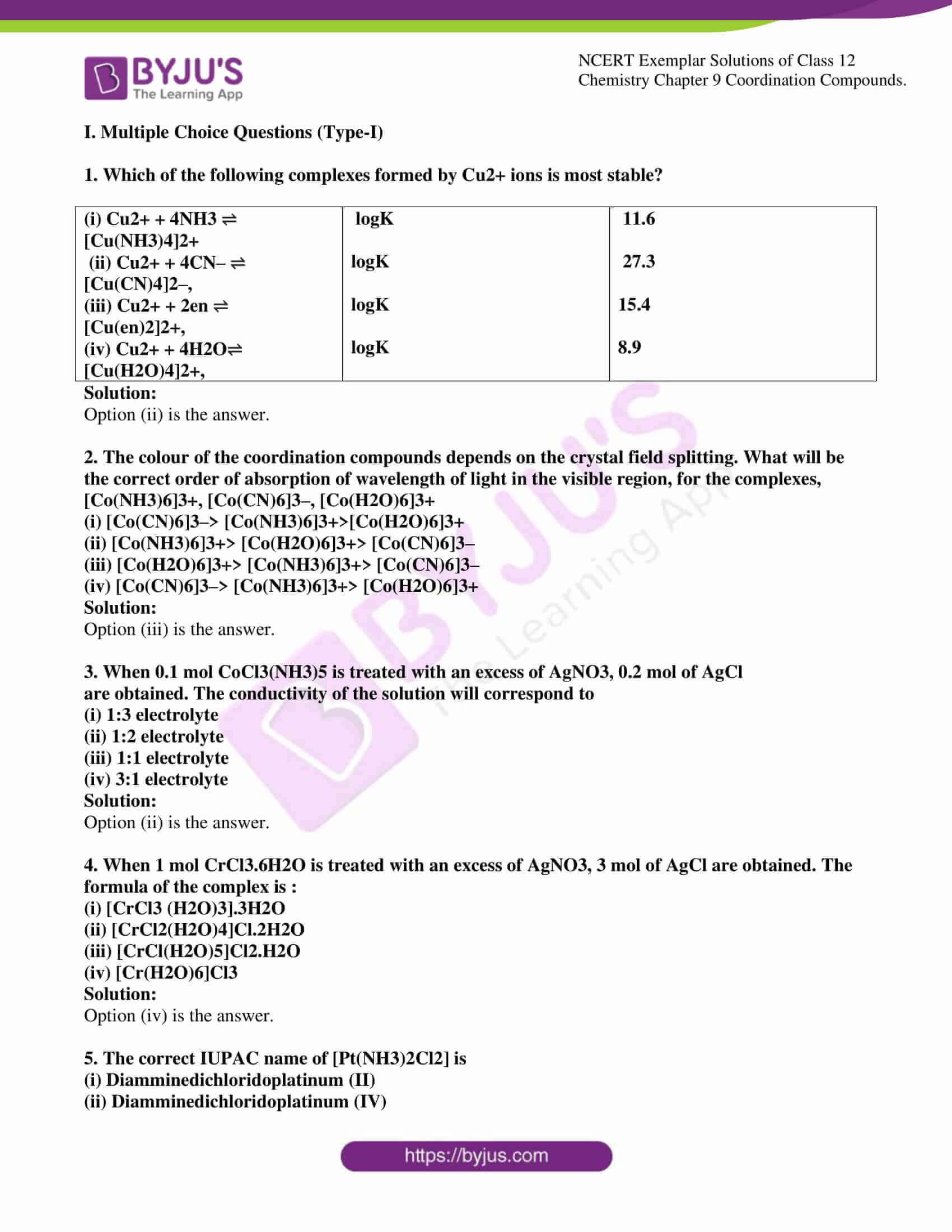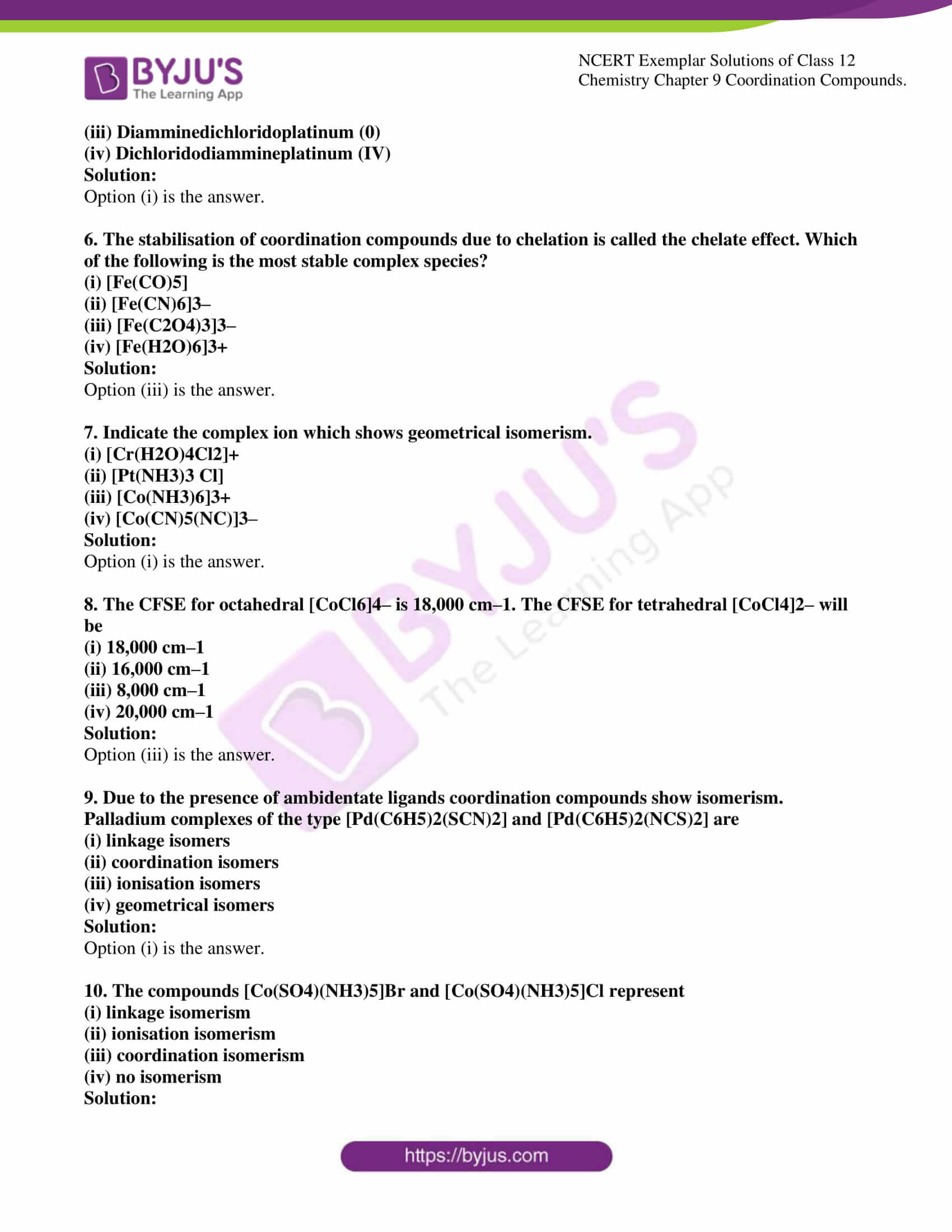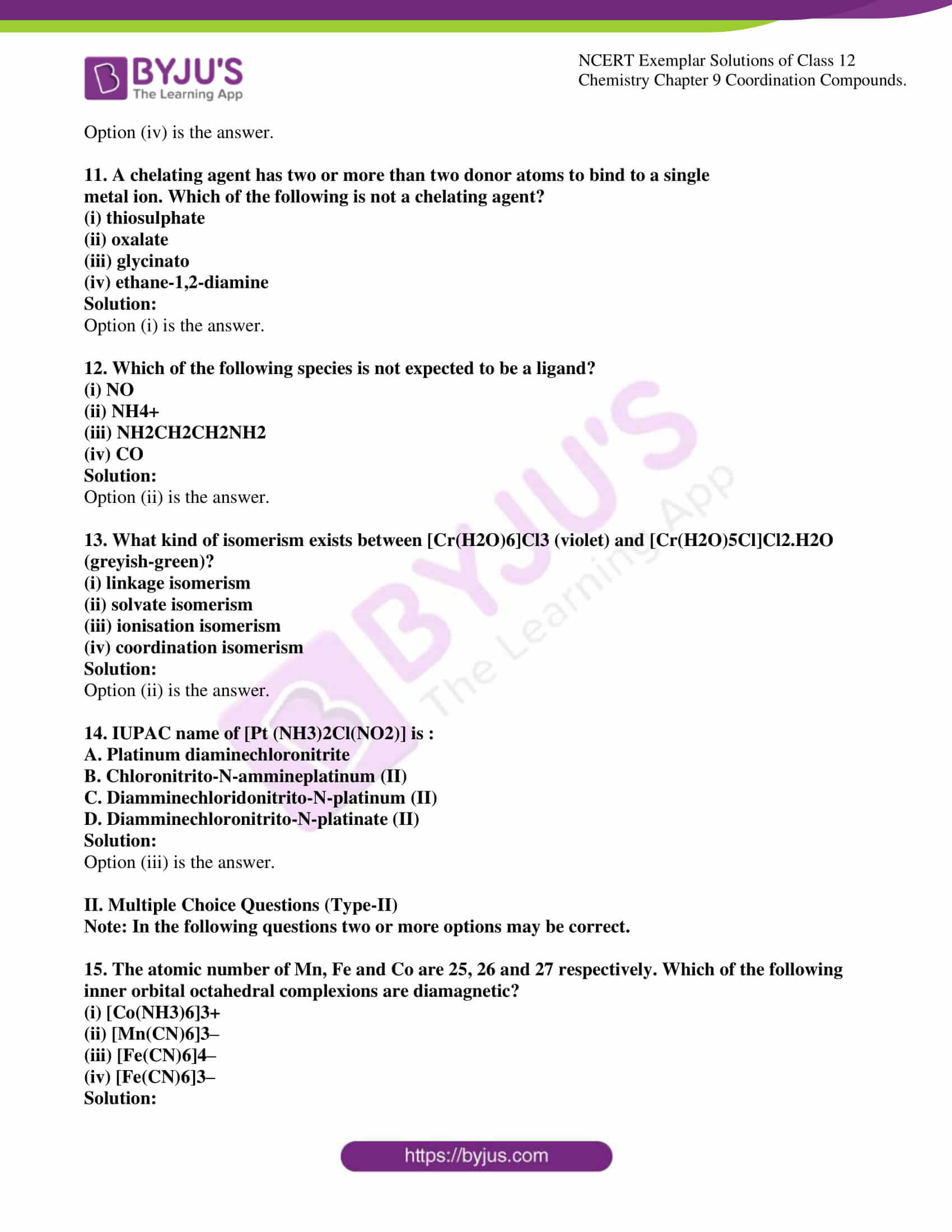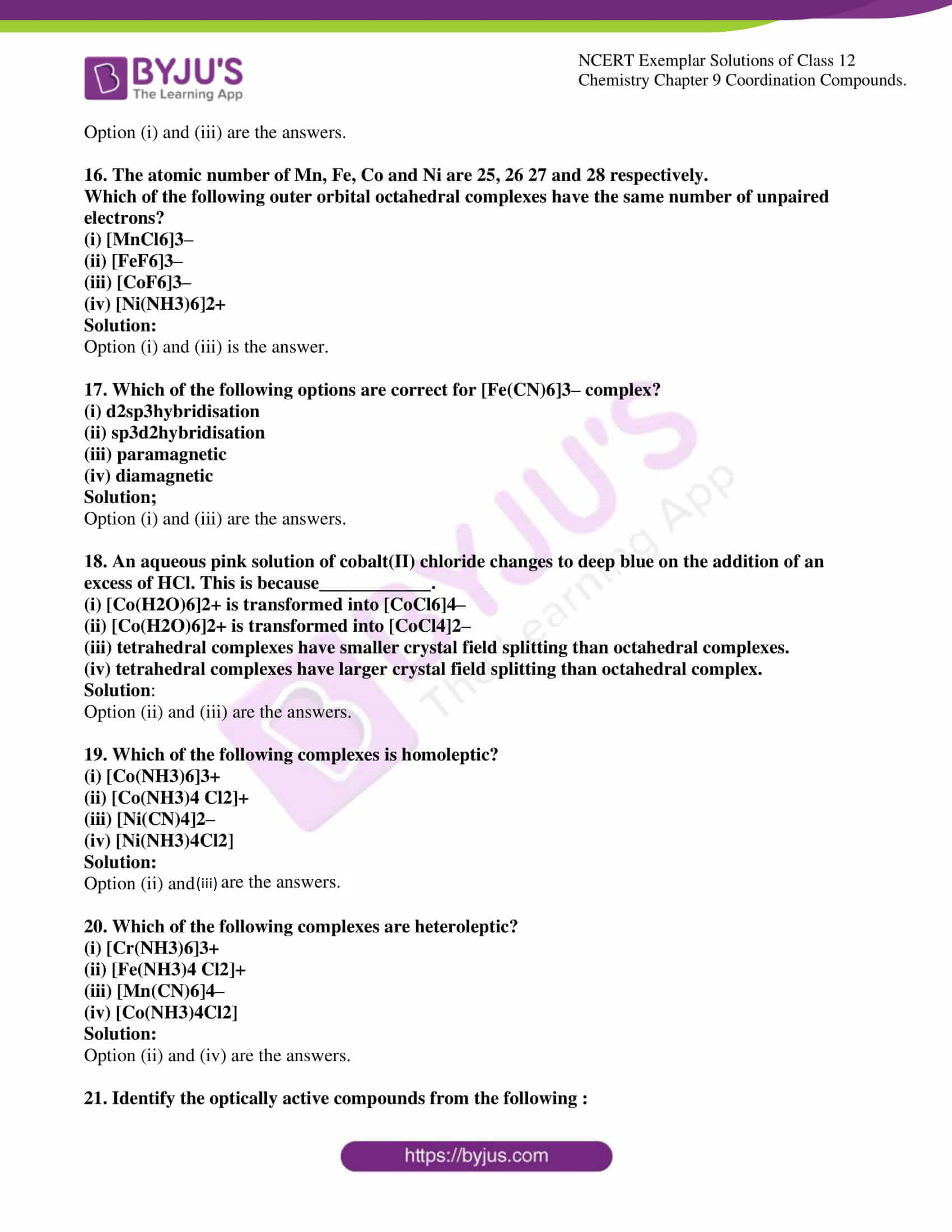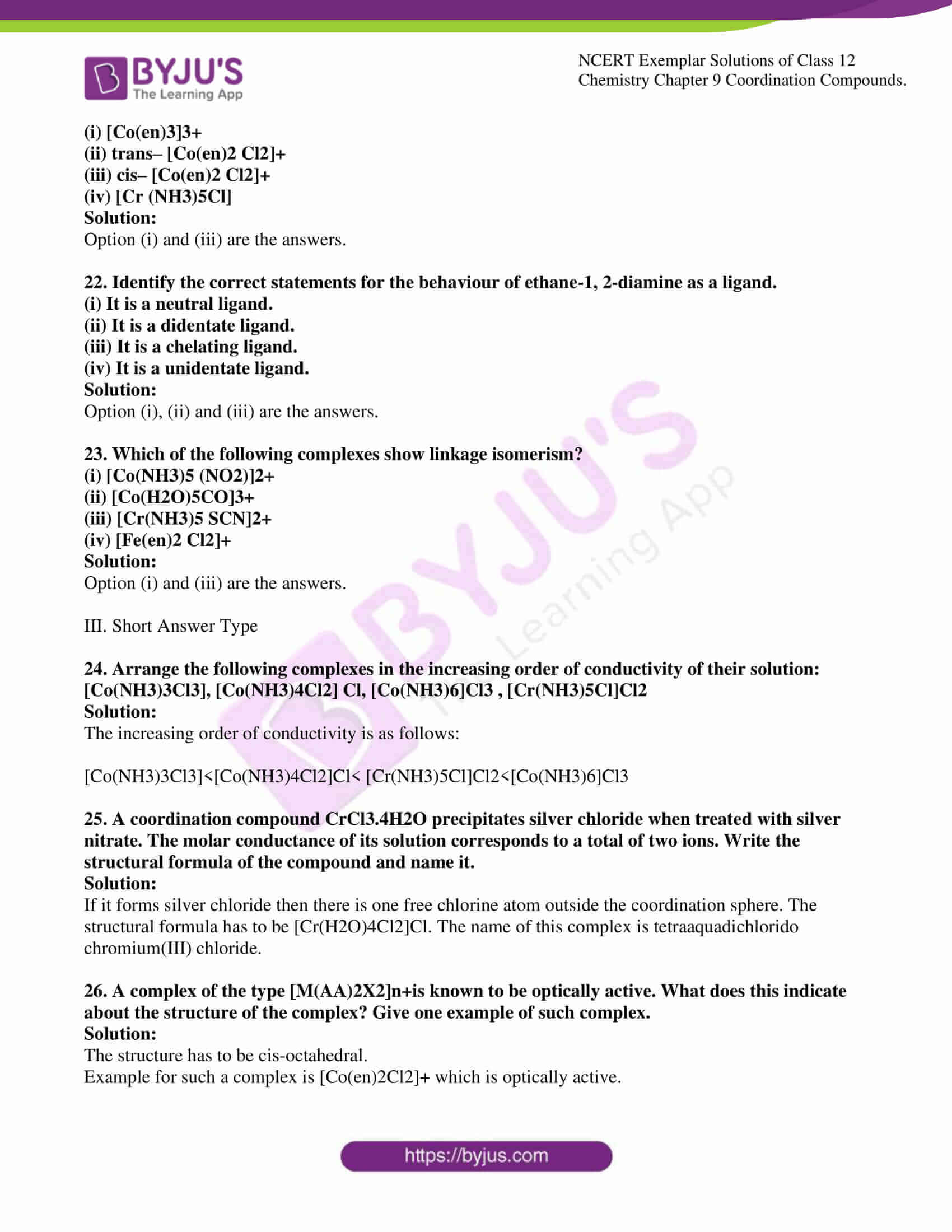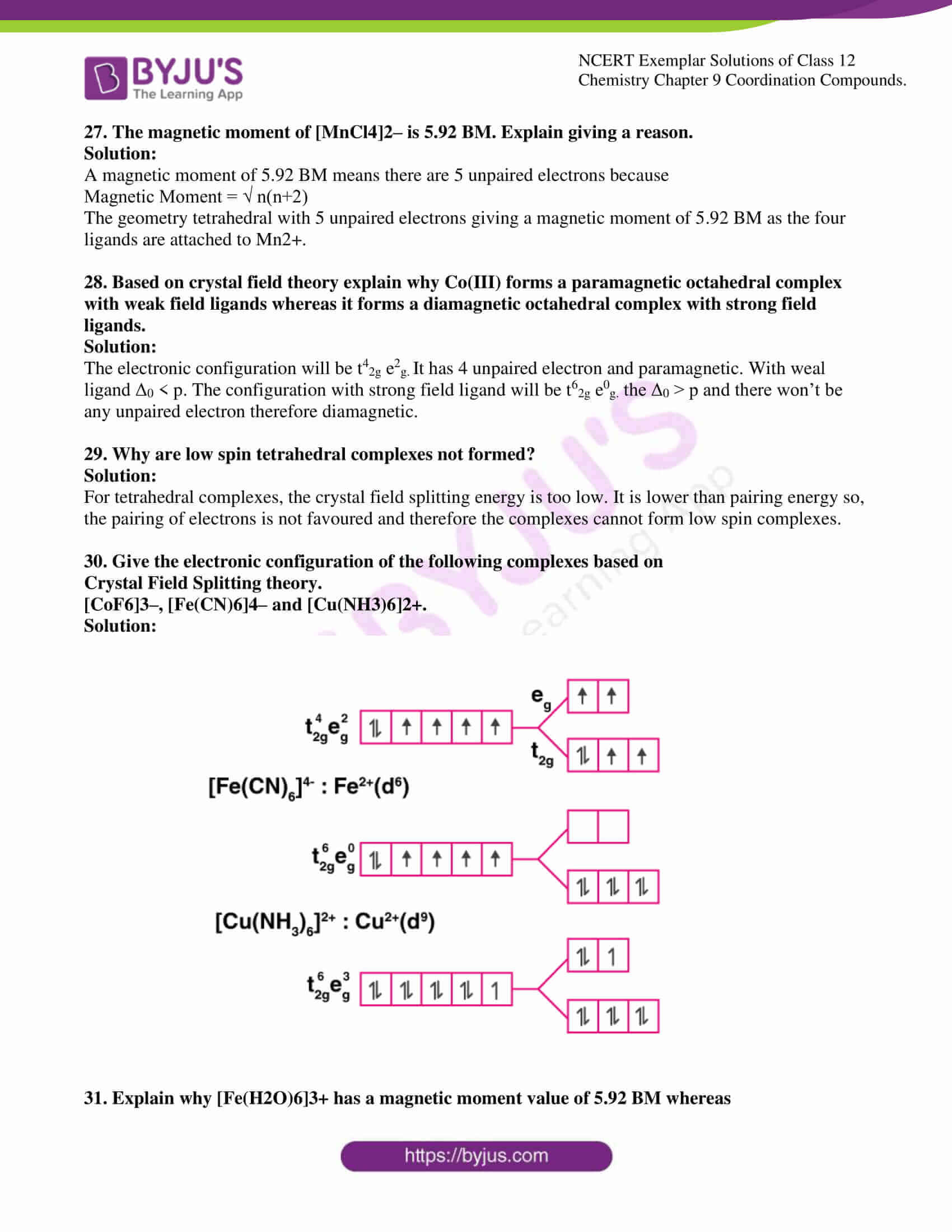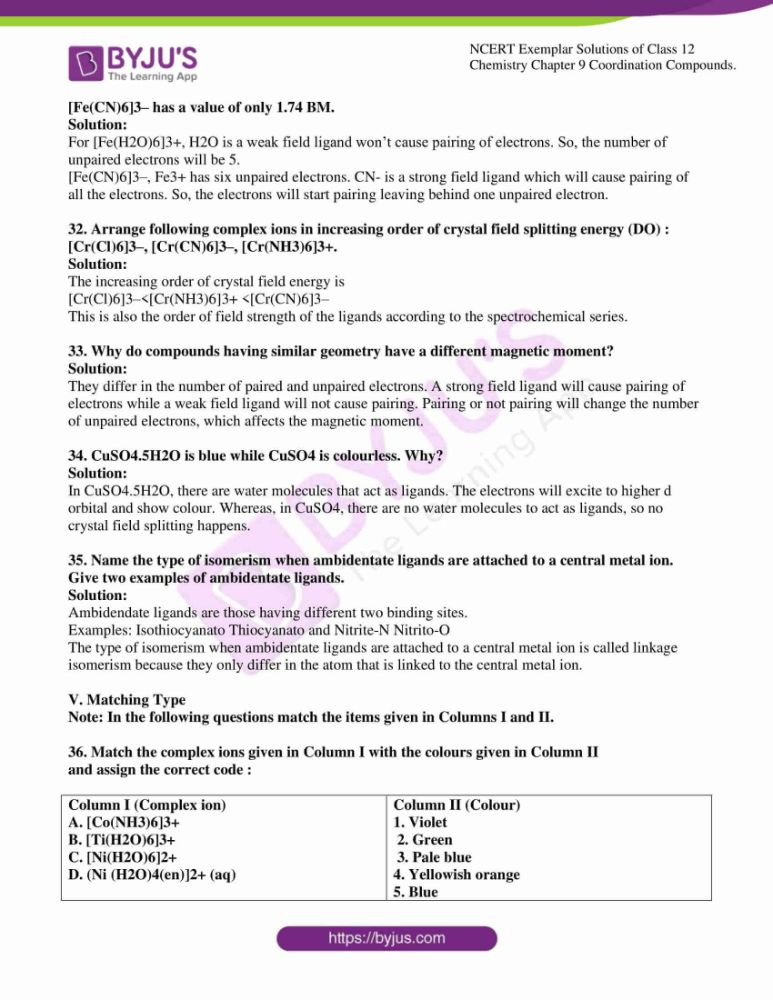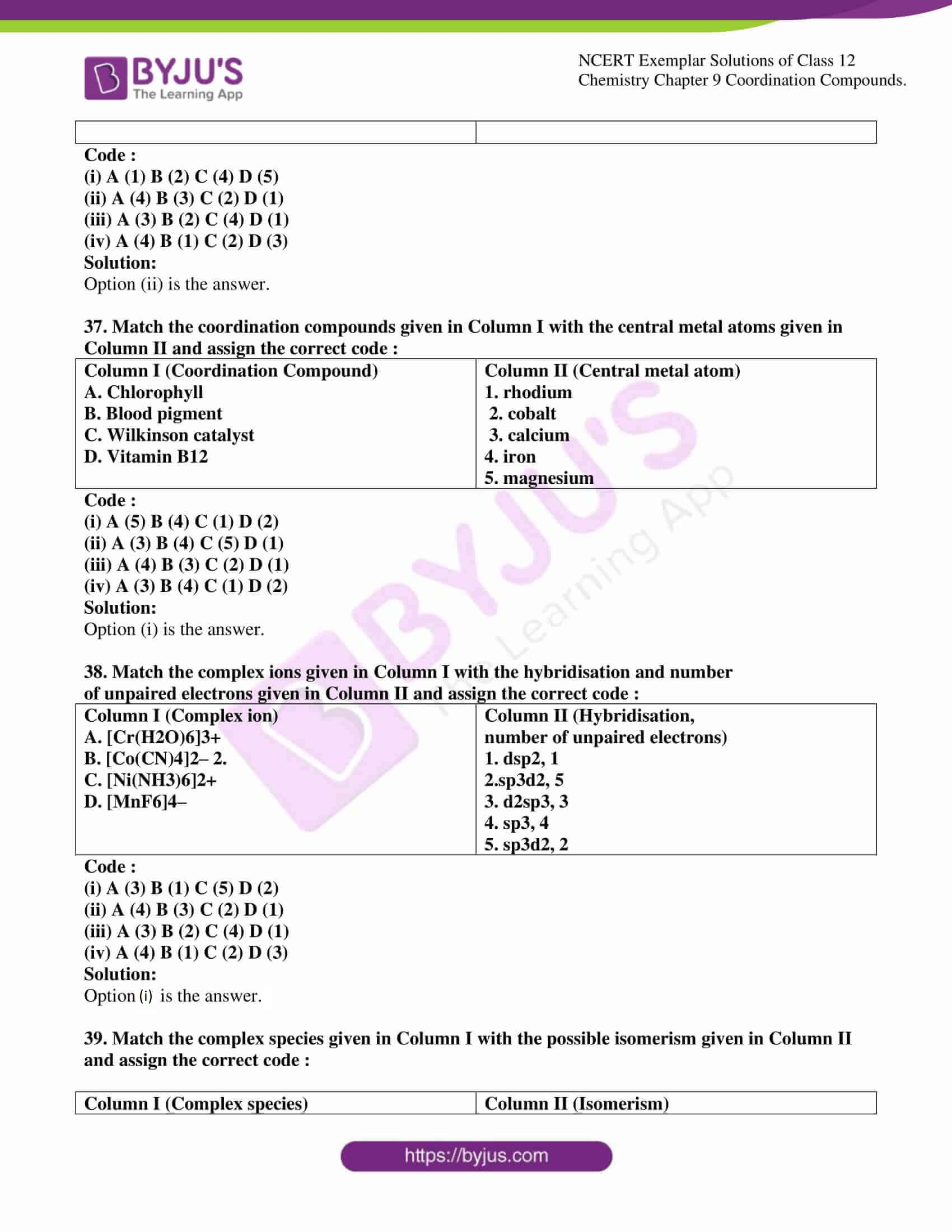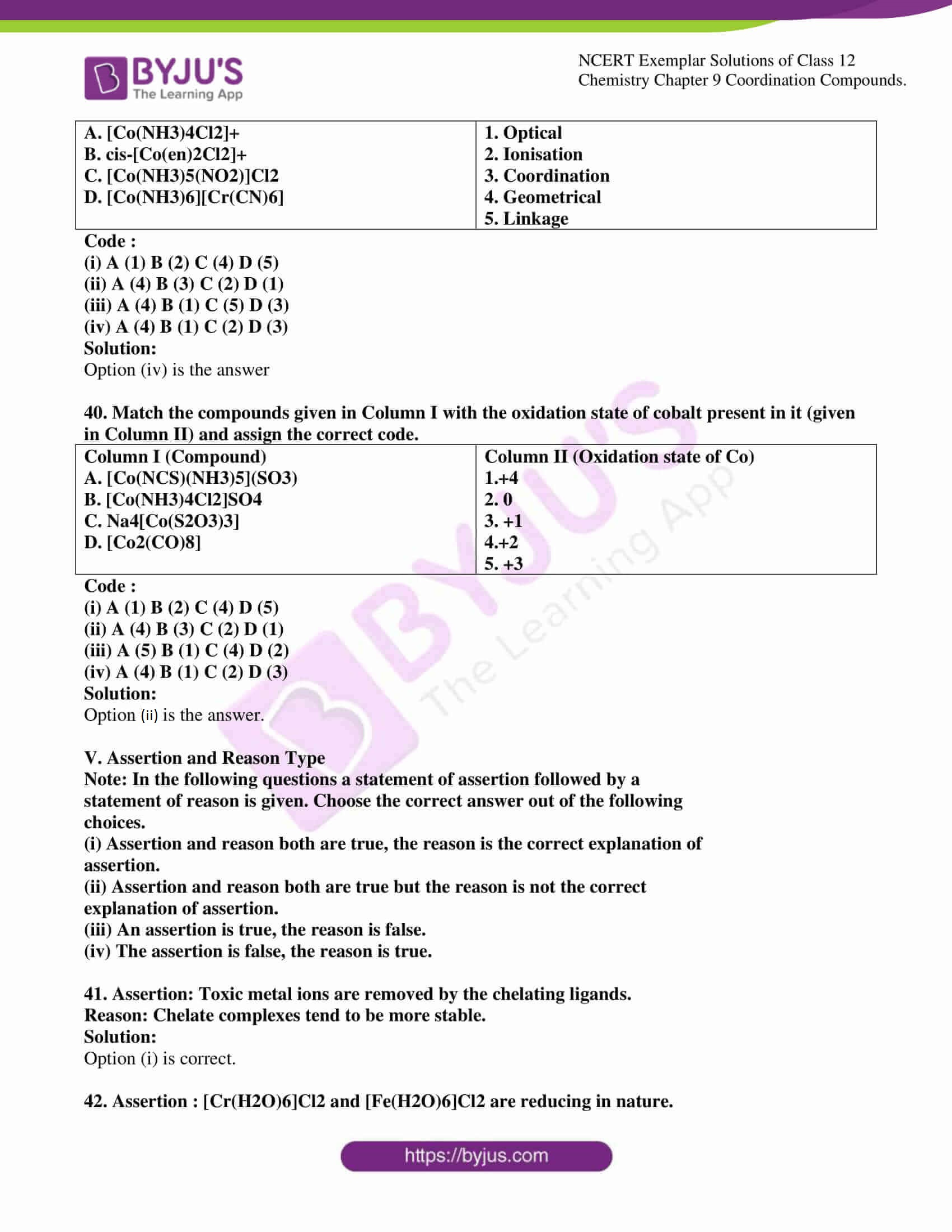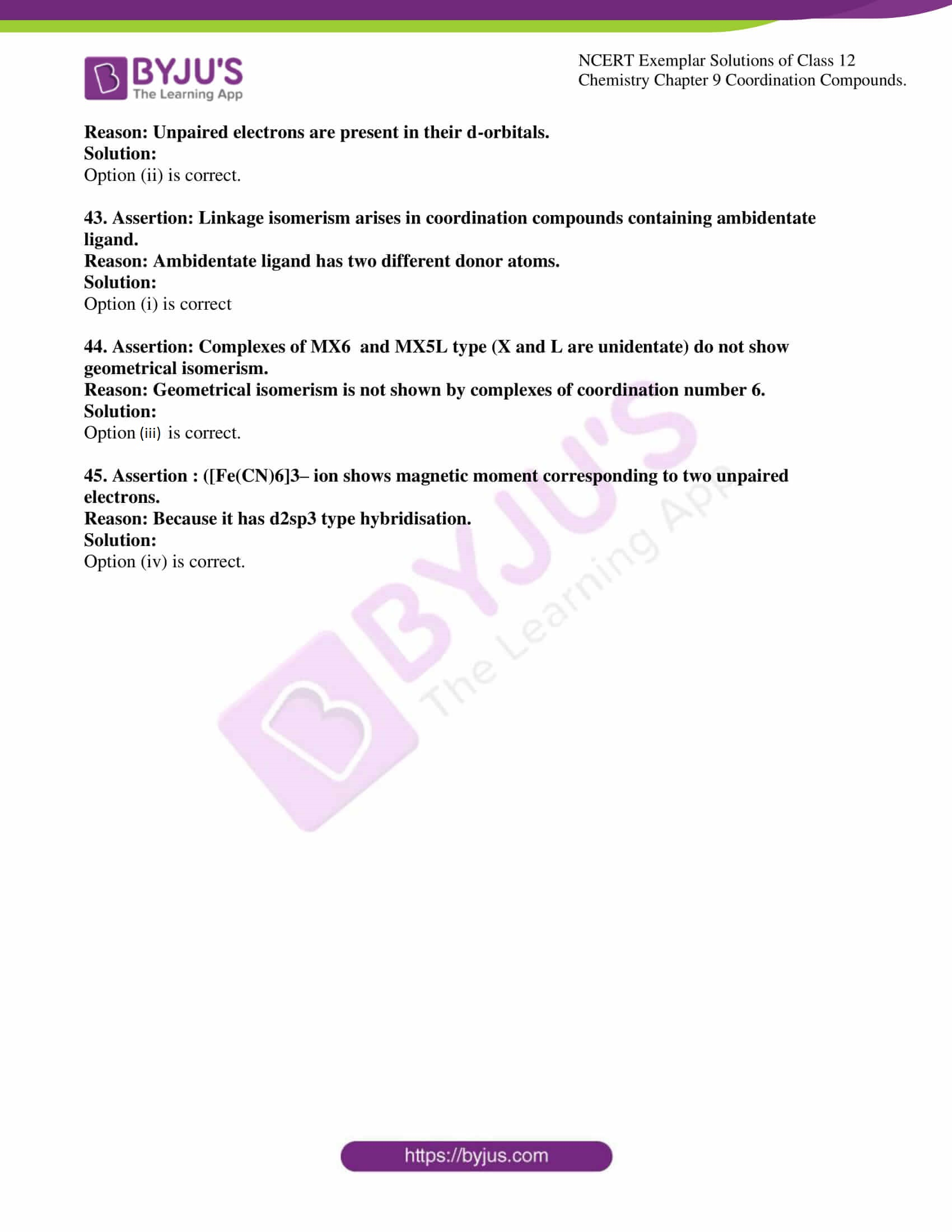### Access Solutions to the NCERT Exemplar Class 12 Chemistry Chapter 9

I. Multiple Choice Questions (Type-I)

1. Which of the following complexes formed by Cu2+ ions is most stable?

 (i) Cu2+ + 4NH3 ⇌ [Cu(NH3)4]2+ (ii) Cu2+ + 4CN– ⇌ [Cu(CN)4]2–, (iii) Cu2+ + 2en ⇌ [Cu(en)2]2+, (iv) Cu2+ + 4H2O⇌ [Cu(H2O)4]2+, logK logK logK logK 11.6 27.3 15.4 8.9

Solution:

2. The colour of the coordination compounds depends on the crystal field splitting. What will be the correct order of absorption of wavelength of light in the visible region, for the complexes, [Co(NH3)6]3+, [Co(CN)6]3–, [Co(H2O)6]3+

(i) [Co(CN)6]3–> [Co(NH3)6]3+>[Co(H2O)6]3+

(ii) [Co(NH3)6]3+> [Co(H2O)6]3+> [Co(CN)6]3–

(iii) [Co(H2O)6]3+> [Co(NH3)6]3+> [Co(CN)6]3–

(iv) [Co(CN)6]3–> [Co(NH3)6]3+> [Co(H2O)6]3+

Solution:

3. When 0.1 mol CoCl3(NH3)5 is treated with an excess of AgNO3, 0.2 mol of AgCl

are obtained. The conductivity of the solution will correspond to

(i) 1:3 electrolyte

(ii) 1:2 electrolyte

(iii) 1:1 electrolyte

(iv) 3:1 electrolyte

Solution:

4. When 1 mol CrCl3.6H2O is treated with an excess of AgNO3, 3 mol of AgCl are obtained. The formula of the complex is :

(i) [CrCl3 (H2O)3].3H2O

(ii) [CrCl2(H2O)4]Cl.2H2O

(iii) [CrCl(H2O)5]Cl2.H2O

(iv) [Cr(H2O)6]Cl3

Solution:

5. The correct IUPAC name of [Pt(NH3)2Cl2] is

(i) Diamminedichloridoplatinum (II)

(ii) Diamminedichloridoplatinum (IV)

(iii) Diamminedichloridoplatinum (0)

(iv) Dichloridodiammineplatinum (IV)

Solution:

6. The stabilisation of coordination compounds due to chelation is called the chelate effect. Which of the following is the most stable complex species?

(i) [Fe(CO)5]

(ii) [Fe(CN)6]3–

(iii) [Fe(C2O4)3]3–

(iv) [Fe(H2O)6]3+

Solution:

7. Indicate the complex ion which shows geometrical isomerism.

(i) [Cr(H2O)4Cl2]+

(ii) [Pt(NH3)3 Cl]

(iii) [Co(NH3)6]3+

(iv) [Co(CN)5(NC)]3–

Solution:

8. The CFSE for octahedral [CoCl6]4– is 18,000 cm–1. The CFSE for tetrahedral [CoCl4]2– will be

(i) 18,000 cm–1

(ii) 16,000 cm–1

(iii) 8,000 cm–1

(iv) 20,000 cm–1

Solution:

9. Due to the presence of ambidentate ligands coordination compounds show isomerism. Palladium complexes of the type [Pd(C6H5)2(SCN)2] and [Pd(C6H5)2(NCS)2] are

(ii) coordination isomers

(iii) ionisation isomers

(iv) geometrical isomers

Solution:

10. The compounds [Co(SO4)(NH3)5]Br and [Co(SO4)(NH3)5]Cl represent

(ii) ionisation isomerism

(iii) coordination isomerism

(iv) no isomerism

Solution:

11. A chelating agent has two or more than two donor atoms to bind to a single

metal ion. Which of the following is not a chelating agent?

(i) thiosulphate

(ii) oxalate

(iii) glycinato

(iv) ethane-1,2-diamine

Solution:

12. Which of the following species is not expected to be a ligand?

(i) NO

(ii) NH4+

(iii) NH2CH2CH2NH2

(iv) CO

Solution:

13. What kind of isomerism exists between [Cr(H2O)6]Cl3 (violet) and [Cr(H2O)5Cl]Cl2.H2O (greyish-green)?

(ii) solvate isomerism

(iii) ionisation isomerism

(iv) coordination isomerism

Solution:

14. IUPAC name of [Pt (NH3)2Cl(NO2)] is :

A. Platinum diaminechloronitrite

B. Chloronitrito-N-ammineplatinum (II)

C. Diamminechloridonitrito-N-platinum (II)

D. Diamminechloronitrito-N-platinate (II)

Solution:

II. Multiple Choice Questions (Type-II)

Note: In the following questions two or more options may be correct.

15. The atomic number of Mn, Fe and Co are 25, 26 and 27 respectively. Which of the following inner orbital octahedral complexions are diamagnetic?

(i) [Co(NH3)6]3+

(ii) [Mn(CN)6]3–

(iii) [Fe(CN)6]4–

(iv) [Fe(CN)6]3–

Solution:

Option (i) and (iii) are the answers.

16. The atomic number of Mn, Fe, Co and Ni are 25, 26 27 and 28 respectively.

Which of the following outer orbital octahedral complexes have the same number of unpaired electrons?

(i) [MnCl6]3–

(ii) [FeF6]3–

(iii) [CoF6]3–

(iv) [Ni(NH3)6]2+

Solution:

Option (i) and (iii) is the answer.

17. Which of the following options are correct for [Fe(CN)6]3– complex?

(i) d2sp3hybridisation

(ii) sp3d2hybridisation

(iii) paramagnetic

(iv) diamagnetic

Solution;

Option (i) and (iii) are the answers.

18. An aqueous pink solution of cobalt(II) chloride changes to deep blue on the addition of an excess of HCl. This is because____________.

(i) [Co(H2O)6]2+ is transformed into [CoCl6]4–

(ii) [Co(H2O)6]2+ is transformed into [CoCl4]2–

(iii) tetrahedral complexes have smaller crystal field splitting than octahedral complexes.

(iv) tetrahedral complexes have larger crystal field splitting than octahedral complex.

Solution:

Option (ii) and (iii) are the answers.

19. Which of the following complexes is homoleptic?

(i) [Co(NH3)6]3+

(ii) [Co(NH3)4 Cl2]+

(iii) [Ni(CN)4]2–

(iv) [Ni(NH3)4Cl2]

Solution:

Option (i) and (iii) are the answers.

20. Which of the following complexes are heteroleptic?

(i) [Cr(NH3)6]3+

(ii) [Fe(NH3)4 Cl2]+

(iii) [Mn(CN)6]4–

(iv) [Co(NH3)4Cl2]

Solution:

Option (ii) and (iv) are the answers.

21. Identify the optically active compounds from the following :

(i) [Co(en)3]3+

(ii) trans– [Co(en)2 Cl2]+

(iii) cis– [Co(en)2 Cl2]+

(iv) [Cr (NH3)5Cl]

Solution:

Option (i) and (iii) are the answers.

22. Identify the correct statements for the behaviour of ethane-1, 2-diamine as a ligand.

(i) It is a neutral ligand.

(ii) It is a didentate ligand.

(iii) It is a chelating ligand.

(iv) It is a unidentate ligand.

Solution:

Option (i), (ii) and (iii) are the answers.

23. Which of the following complexes show linkage isomerism?

(i) [Co(NH3)5 (NO2)]2+

(ii) [Co(H2O)5CO]3+

(iii) [Cr(NH3)5 SCN]2+

(iv) [Fe(en)2 Cl2]+

Solution:

Option (i) and (iii) are the answers.

24. Arrange the following complexes in the increasing order of conductivity of their solution: [Co(NH3)3Cl3], [Co(NH3)4Cl2] Cl, [Co(NH3)6]Cl3 , [Cr(NH3)5Cl]Cl2

Solution:

The increasing order of conductivity is as follows:

[Co(NH3)3Cl3]<[Co(NH3)4Cl2]Cl< [Cr(NH3)5Cl]Cl2<[Co(NH3)6]Cl3

25. A coordination compound CrCl3.4H2O precipitates silver chloride when treated with silver nitrate. The molar conductance of its solution corresponds to a total of two ions. Write the structural formula of the compound and name it.

Solution:

If it forms silver chloride then there is one free chlorine atom outside the coordination sphere. The structural formula has to be [Cr(H2O)4Cl2]Cl. The name of this complex is tetraaquadichlorido chromium(III) chloride.

26. A complex of the type [M(AA)2X2]n+is known to be optically active. What does this indicate about the structure of the complex? Give one example of such complex.

Solution:

The structure has to be cis-octahedral.

Example for such a complex is [Co(en)2Cl2]+ which is optically active.

27. The magnetic moment of [MnCl4]2– is 5.92 BM. Explain giving a reason.

Solution:

A magnetic moment of 5.92 BM means there are 5 unpaired electrons because

Magnetic Moment = √ n(n+2)

The geometry tetrahedral with 5 unpaired electrons giving a magnetic moment of 5.92 BM as the four ligands are attached to Mn2+.

28. Based on crystal field theory explain why Co(III) forms a paramagnetic octahedral complex with weak field ligands whereas it forms a diamagnetic octahedral complex with strong field ligands.

Solution:

The electronic configuration will be t42g e2g. It has 4 unpaired electron and paramagnetic. With weal ligand Δ0 < p. The configuration with strong field ligand will be t62g e0g. the Δ0 > p and there won’t be any unpaired electron therefore diamagnetic.

29. Why are low spin tetrahedral complexes not formed?

Solution:

For tetrahedral complexes, the crystal field splitting energy is too low. It is lower than pairing energy so, the pairing of electrons is not favoured and therefore the complexes cannot form low spin complexes.

30. Give the electronic configuration of the following complexes based on

Crystal Field Splitting theory.

[CoF6]3–, [Fe(CN)6]4– and [Cu(NH3)6]2+.

Solution: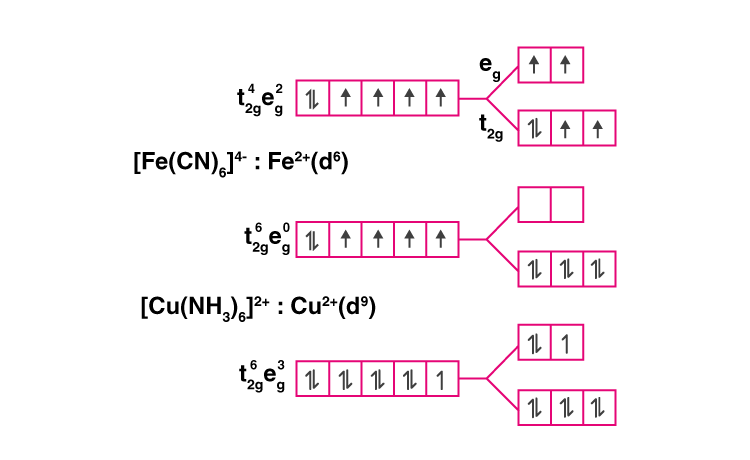31. Explain why [Fe(H2O)6]3+ has a magnetic moment value of 5.92 BM whereas

[Fe(CN)6]3– has a value of only 1.74 BM.

Solution:

For [Fe(H2O)6]3+, H2O is a weak field ligand won’t cause pairing of electrons. So, the number of unpaired electrons will be 5.

[Fe(CN)6]3–, Fe3+ has six unpaired electrons. CN- is a strong field ligand which will cause pairing of all the electrons. So, the electrons will start pairing leaving behind one unpaired electron.

32. Arrange following complex ions in increasing order of crystal field splitting energy (DO) :

[Cr(Cl)6]3–, [Cr(CN)6]3–, [Cr(NH3)6]3+.

Solution:

The increasing order of crystal field energy is

[Cr(Cl)6]3–<[Cr(NH3)6]3+ <[Cr(CN)6]3–

This is also the order of field strength of the ligands according to the spectrochemical series.

33. Why do compounds having similar geometry have a different magnetic moment?

Solution:

They differ in the number of paired and unpaired electrons. A strong field ligand will cause pairing of electrons while a weak field ligand will not cause pairing. Pairing or not pairing will change the number of unpaired electrons, which affects the magnetic moment.

34. CuSO4.5H2O is blue while CuSO4 is colourless. Why?

Solution:

In CuSO4.5H2O, there are water molecules that act as ligands. The electrons will excite to higher d orbital and show colour. Whereas, in CuSO4, there are no water molecules to act as ligands, so no crystal field splitting happens.

35. Name the type of isomerism when ambidentate ligands are attached to a central metal ion. Give two examples of ambidentate ligands.

Solution:

Ambidendate ligands are those having different two binding sites.

Examples: Isothiocyanato Thiocyanato and Nitrite-N Nitrito-O

The type of isomerism when ambidentate ligands are attached to a central metal ion is called linkage isomerism because they only differ in the atom that is linked to the central metal ion.

V. Matching Type

Note: In the following questions match the items given in Columns I and II.

36. Match the complex ions given in Column I with the colours given in Column II

and assign the correct code :

 Column I (Complex ion) A. [Co(NH3)6]3+ B. [Ti(H2O)6]3+ C. [Ni(H2O)6]2+ D. (Ni (H2O)4(en)]2+ (aq) Column II (Colour) 1. Violet 2. Green 3. Pale blue 4. Yellowish orange 5. Blue

Code :

(i) A (1) B (2) C (4) D (5)

(ii) A (4) B (3) C (2) D (1)

(iii) A (3) B (2) C (4) D (1)

(iv) A (4) B (1) C (2) D (3)

Solution:

37. Match the coordination compounds given in Column I with the central metal atoms given in Column II and assign the correct code :

 Column I (Coordination Compound) A. Chlorophyll B. Blood pigment C. Wilkinson catalyst D. Vitamin B12 Column II (Central metal atom) 1. rhodium 2. cobalt 3. calcium 4. iron 5. magnesium

Code :

(i) A (5) B (4) C (1) D (2)

(ii) A (3) B (4) C (5) D (1)

(iii) A (4) B (3) C (2) D (1)

(iv) A (3) B (4) C (1) D (2)

Solution:

38. Match the complex ions given in Column I with the hybridisation and number

of unpaired electrons given in Column II and assign the correct code :

 Column I (Complex ion) A. [Cr(H2O)6]3+ B. [Co(CN)4]2– 2. C. [Ni(NH3)6]2+ D. [MnF6]4– Column II (Hybridisation, number of unpaired electrons) 1. dsp2, 1 2.sp3d2, 5 3. d2sp3, 3 4. sp3, 4 5. sp3d2, 2

Code :

(i) A (3) B (1) C (5) D (2)

(ii) A (4) B (3) C (2) D (1)

(iii) A (3) B (2) C (4) D (1)

(iv) A (4) B (1) C (2) D (3)

Solution:

39. Match the complex species given in Column I with the possible isomerism given in Column II and assign the correct code :

 Column I (Complex species) A. [Co(NH3)4Cl2]+ B. cis-[Co(en)2Cl2]+ C. [Co(NH3)5(NO2)]Cl2 D. [Co(NH3)6][Cr(CN)6] Column II (Isomerism) 1. Optical 2. Ionisation 3. Coordination 4. Geometrical 5. Linkage

Code :

(i) A (1) B (2) C (4) D (5)

(ii) A (4) B (3) C (2) D (1)

(iii) A (4) B (1) C (5) D (3)

(iv) A (4) B (1) C (2) D (3)

Solution:

40. Match the compounds given in Column I with the oxidation state of cobalt present in it (given in Column II) and assign the correct code.

 Column I (Compound) A. [Co(NCS)(NH3)5](SO3) B. [Co(NH3)4Cl2]SO4 C. Na4[Co(S2O3)3] D. [Co2(CO)8] Column II (Oxidation state of Co) 1.+4 2. 0 3. +1 4.+2 5. +3

Code :

(i) A (1) B (2) C (4) D (5)

(ii) A (4) B (3) C (2) D (1)

(iii) A (5) B (1) C (4) D (2)

(iv) A (4) B (1) C (2) D (3)

Solution:

V. Assertion and Reason Type

Note: In the following questions a statement of assertion followed by a

statement of reason is given. Choose the correct answer out of the following

choices.

(i) Assertion and reason both are true, the reason is the correct explanation of

assertion.

(ii) Assertion and reason both are true but the reason is not the correct

explanation of assertion.

(iii) An assertion is true, the reason is false.

(iv) The assertion is false, the reason is true.

41. Assertion: Toxic metal ions are removed by the chelating ligands.

Reason: Chelate complexes tend to be more stable.

Solution:

Option (i) is correct.

42. Assertion : [Cr(H2O)6]Cl2 and [Fe(H2O)6]Cl2 are reducing in nature.

Reason: Unpaired electrons are present in their d-orbitals.

Solution:

Option (ii) is correct.

43. Assertion: Linkage isomerism arises in coordination compounds containing ambidentate ligand.

Reason: Ambidentate ligand has two different donor atoms.

Solution:

Option (i) is correct

44. Assertion: Complexes of MX6 and MX5L type (X and L are unidentate) do not show geometrical isomerism.

Reason: Geometrical isomerism is not shown by complexes of coordination number 6.

Solution:

Option (iii) is correct.

45. Assertion : ([Fe(CN)6]3– ion shows magnetic moment corresponding to two unpaired electrons.

Reason: Because it has d2sp3 type hybridisation.

Solution:

Option (iv) is correct.

### Class 12 Chemistry NCERT Exemplar Problems for Coordination Compounds

Coordination Compounds is one of the most interesting and easy chapters in the CBSE Class 12 Chemistry. In this chapter, students get to learn and understand the terms like central ion, atom, ligand, coordination sphere, coordination polyhedron and oxidation number. They will also learn the rules of the nomenclature of coordination compounds.

#### Important Concepts to Study in Chapter 9 Coordination Compounds

1. Werner’s Theory of Coordination Compounds
2. Definitions of Some Important Terms Pertaining to Coordination Compounds
3. Nomenclature of Coordination Compounds
4. Isomerism in Coordination Compounds
5. Bonding in Coordination Compounds
6. Bonding in Metal Carbonyls
7. Stability of Coordination Compounds
8. Importance and Applications of Coordination Compounds.

To help students score good marks in exams, we have provided the NCERT Exemplar Class 12 Chemistry Chapter 9 Coordination Compounds.

### Why Opt for BYJU’S

Apart from the NCERT Class 12 Chemistry Exemplar for Chapter 9, get chapter-wise NCERT Exemplars for Class 12 Physics, Maths and Biology. Students can download worksheets, assignments, NCERT Class 12 Science Solution and other study materials for exam preparation and score excellent marks.